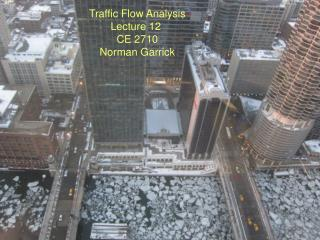Download PresentationTraffic Flow Analysis Lecture 12 CE 2710 Norman Garrick# Traffic Flow Analysis Lecture 12 CE 2710 Norman Garrick - PowerPoint PPT Presentation

Download Presentation##### Traffic Flow Analysis Lecture 12 CE 2710 Norman Garrick

Download Policy: Content on the Website is provided to you AS IS for your information and personal use and may not be sold / licensed / shared on other websites without getting consent from its author. While downloading, if for some reason you are not able to download a presentation, the publisher may have deleted the file from their server.

- - - - - - - - - - - - - - - - - - - - - - - - - - - E N D - - - - - - - - - - - - - - - - - - - - - - - - - - -
##### Presentation Transcript

1. Traffic Flow Analysis Lecture 12 CE 2710 Norman Garrick Norman W. Garrick

2. Traffic Flow Patterns of the Future? http://www.youtube.com/watch?v=KX46uhpAQaw European SATRE Car Platoon Road Test Norman W. Garrick

3. Basic Parameters for Understanding Stream Flow Three basic classes of parameters Spacing and Concentration Headway and Flow Speed Norman W. Garrick

4. s1 s2 s3 s4 Spacing and Concentration D Spacing is the distance between vehicles, s Concentration (or density) is the number of vehicles per unit length, k s(ave) = 1 / k Norman W. Garrick

5. Spacing and ConcentrationExample D s1 s2 s3 s4 If D = 1/10 mile What is the concentration, k? We have 4 vehicles in 1/10 mile Therefore, the concentration is 40 vehicles/mile What is the average spacing, s? s = 1 / k= 1 / 40 = 0.025 miles = 132 feet Norman W. Garrick

6. s1 s2 s3 s4 Spacing Velocity Spacing and Speed? D ? Norman W. Garrick

7. s1 s2 s3 s4 Spacing Velocity Spacing and Speed? D Space between vehicles increases with speed The relationship between space and speed is not exact It depends on the individual driver, cultural factors, weather etc. Norman W. Garrick

8. Traffic Spacing in India Norman W. Garrick

9. Headway and Flow measurement point Headway is the time between vehicles passing a specific location, h Flow is the amount of vehicles passing a specific location in a specific time, q h(ave) = 1 / q Norman W. Garrick

10. Headway and FlowExample measurement point If the total time for all the vehicles to cross the measurement points is 1/20 hour, what is the flow per hour? 5 vehicles cross in 1/20 hour Flow, q = 100 vehicles per hour What is the average headway? h = 1/q = 1/100 = 0.01 hour = 36 sec Norman W. Garrick

11. Space Parameters and Time Parameters? Is there a relationship between the space parameters and the time parameters? What links these two parameters? Speed! Norman W. Garrick

12. Measuring Average or Mean Speed The average speed of vehicles in a traffic stream can be measured in a number of different ways. Two common methods are used in transportation characterization Time Mean Speed - is based on the spot speed of the vehicles passing a specific point Space Mean Speed - is based on the average time it takes vehicles to cover a certain specified distance Different traffic flow models use different definition of speed – some TMS and some SMS Norman W. Garrick

13. Time Mean Speed is the Arithmetic Average of the Spot Speeds measurement point v= 10 mph v = 15 mph v = 7.5 mph Time Mean Speed,ut = 1/n ∑ vi = (10 + 15 + 7.5) / 3 = 10.8 mph Norman W. Garrick

14. Space Mean Speed D = 1/2 mile t= 3 min start point end point t = 2 min t = 4 min Space Mean Speed is based on the average time t(ave) = (t1 + t2 + t3) / n = (3 + 2 + 4) / 3 = 3 minutes = 1/20 hour Space mean speed, us = D / t(ave) = (1/2) / (1/20) = 10 mph Average speed measured by TMS and SMS is different even though the individual bike speed are exactly the same Norman W. Garrick

15. TMS versus SMS v= 10 mph v = 15 mph v = 7.5 mph Average speed measured by TMS and SMS is different even though the individual bike speed are exactly the same Time Mean Speed,ut = 1/n ∑ vi Space mean speed,us = D / t(ave) Norman W. Garrick

16. SMS v= 10 mph v = 15 mph v = 7.5 mph • Space mean speed,us = D / t(ave) • but, t(ave) = 1/n∑ ti • and, vi= D/ti • >>> ti= D/vi • therefore, us= D / {1/n∑ D/vi } • = D / {D/n ∑ 1/vi } • = 1 / {1/n ∑ 1/vi } Norman W. Garrick

17. SMS is the Harmonic Average of the Spot Speeds v= 10 mph v = 15 mph v = 7.5 mph Space mean speed,us = D / t(ave) = 1 / {1/n ∑ 1/vi } = 1 / {(1/3)*[(1/10)+(1/15)+(1/7.5)]} = 10 mph Norman W. Garrick

18. TMS and SMS Time Mean Speed - is based on the spot speed of the vehicles passing a specific point Space Mean Speed - is based on the average time it takes vehicles to cover a certain specified distance Time Mean Speed,ut = 1/n ∑ vi Space mean speed,us = 1 / {1/n ∑ 1/vi } Norman W. Garrick

19. Relationship between Space and Time Parameters D = 1 mile 100 vehicles What is the concentration? k = 100 vehicles/mile What is the space? s = 1/k = 1/100 miles = 52.8 feet If the average speed = 10 miles What is the flow (q)? Flow is total number of vehicles passing any point Flow = 10*100 = 1000 vehicles/hr In other words, q = uk Norman W. Garrick